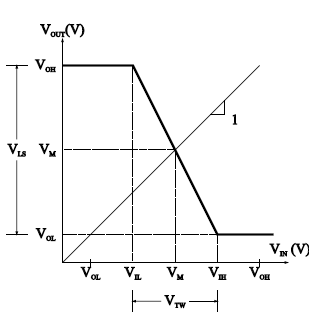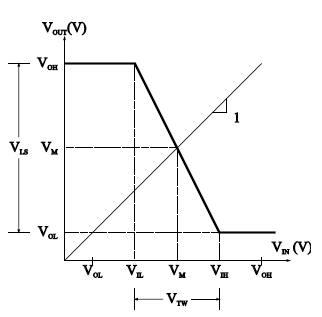### Characteristics of pressure vessels

Finite element method is a method of solving a problem by dividing the problem in to small problems by dividing or breaking up the geometric model into small pieces that are easier to analyze called discretization. In the case of the cylinder it might be broken up into small cubes or plates called elements. All of the elements make up the mesh. Each of these elements can be easily solved for by using simple equations for stress and strain. As the number of elements increases (increasing mesh size), their size decreases and the solution will grow more accurate.
An excellent comparison to finite element analysis is the solution for area under the curve. If one is trying to find the area under the curve, breaking the curve up into a series of rectangles and adding their areas can approximate the solution. The no of rectangular divisions increase the accuracy.
With the recent increases in the speed and processing power of computers, finite element analysis has become a practical way to solve many problems. The increase in computer capabilities has allowed for even greater numbers of elements and higher order elements to be used so that calculated solutions will be fairly accurate.
Unfortunately, due to incorrect meshing and constraining, the structure does not simulate the actual structure being analyzed. Depending on the subject being modelled the method used can become extremely important.
One application of finite element analysis is for pressure vessels. The pressure vessels may be different types. Domestic gas cylinder is one made in two half and welded at centre. Other types are cylinders with separate cap at ends.
There are several ways to model pressure vessels using finite element analysis. If modelled correctly these methods should result in the same solution, but the time spent in calculating solutions can be different. If the finite element analysis solution has an error of five percent and takes an hour to run, that solution may be considered acceptable when compared to a solution with a one percent error that takes twenty hours to run depending on the particular use of the structure and factor of safety. So element selection, approach and size of elements used are much critical during analysis.

### Inverter and Noise in Digital Circuits

Inverter – Voltage Transfer Characteristic
Voltage Transfer Characteristic (VTC) for logic inverters have been standardized.
VTC is the graph between Vout and VIN. On vertical axis, VOH and VOL correspond to output high and
output low voltage levels respectively. On the horizontal axis, VIL is input low voltage and VIH is the input high voltage.

Inverter – Voltage Transfer Characteristic
As the input voltage is increased from 0V, VIL is the maximum input voltage that provides a high output voltage (logical 1 output).
VIH is the minimum input voltage that provides a low output voltage (logical 0 output). VOH, VOL, VIL and VIH are referred to as the critical voltages of the VTC.
VOH > VIH
VOL < VIL

Midpoint Voltage:
Sometimes referred as Threshold voltage  (Vth).
The voltage at which Vout = VIN on VTC is referred as Midpoint voltage. Midpoint voltage can be found graphically by superimposing (the unity slope) Vout = VIN and finding its intersection with the VTC

Logic Swing and Transition Width
Logic Swing
The magnitude of voltage difference between the output high and low voltage levels.
VLS = VOH – VOL

Transition Width
The amount of voltage change that is required of the input voltage to cause a change in the output voltage from the high to the low level (and vice versa).
VTW = VIH - VIL

Noise in Digital Circuits
Noise
Variations in the steady-state voltage levels of digital circuits (i.e. Logical 1 and logical 0 states) are undesirable and cause logic errors. This variation is termed as Noise.

Noise Margins
Voltage Noise Margin represents the safety margin for the high and low voltage levels. Noise voltages must have magnitudes less than the voltage noise margins.
VNMH = VOH – VIH
VNML = VIL - VOL

Noise Sensitivities
The effects of input variations are quantified in terms of the noise sensitivities.
The high noise sensitivity is defined as the difference between input and midpoint voltage for VIN  at VOH.
The low noise sensitivity is defined as the difference between input and midpoint voltage for VIN at VOL.
VNSH = VOH – VM
VNSL = VM - VOL

Noise Immunities
The ability of a gate to reject noise. The high and low noise immunities are defined as the quotient of the noise sensitivities and the logic swing.
VNIH = VNSH / VLS
VNIL = VNSL / VLS

FAN-IN and FAN-OUT
A general logic gate has multiple inputs and multiple outputs.
By multiple outputs we mean the output of a given gate is connected to (driving) the inputs of several load gates.
FAN-IN: the number of inputs of a gate.
FAN-OUT: the number of outputs of a gate.
Maximum FAN-OUT depends on the input and output current of a driving gate.
The maximum fan-out possible during the driving gate’s logical 1 output state is
Nhigh = IOUT (high)/ I’IN (high)
The maximum fan-out possible during the driving gate’s logical 0 output state is
Nlow = IOUT (low)/ I’IN (low)

### Finite Elemental Analysis

Finite elemental analysis refers to a new method of analyzing various parameters of a given structure such as the structural stiffness, thermal behavior and fluid flow interaction. The method is one of approximation and is undergoing tremendous development, with the introduction of newer algorithms and improved computing capability. Today’s industrial scenario demands that any technical solution provided should be minimal in terms of both the product development time and the cost. This has given rise to newer methods of analysis
which do not involve the production of a prototype. Owing to its sustained credibility, FEA has become one of the most widely used analysis tools. The first part of the assignment is concerned with a debate with regard to the merits
and demerits of empirical methods as against the FEA method. This section deals with an overview of the finite elemental method procedure and its related merits. The second section deals with assessing the structural behavior of the structure, in terms of stresses, displacement etc. The same parameters have been calculated manually and have been compared. The reason for discrepancies, if any has been assessed. The third section deals with conducting a thermal analysis of the pressure vessel. This is important, as it provides a clear understanding of the thermal stresses being generated and the temperature distribution. The outcomes of the assignment included getting a clear picture of the role of Finite elemental method in the product life cycle and understanding the significance of industry increasingly favoring the usage of tools such as finite elemental analysis over empirical approach. The procedures for determining the behavior of a structure were also learn.

The term empirical is defined as ‘Relying on or derived from observation or experiment. The origin of all forms of science can be traced to the keenness of man to understand his surroundings. Right from man’s understanding of the presence of light to the motion of the planets, human understanding has been based on his observation of the world around him. His understanding of the world has also been fueled by his attempts to mimic nature. Hence it can be safely said that the basis of all science has been human observation. Finite Elemental Method [FEM] is an approximation method used to determine the properties of a structure by solving suitable mathematical models in an iterative fashion. FEA refers to the analysis method, which employs FEM. ‘Checking the designed object for its functionality is called as engineering analysis.’ The process is more resource intensive in comparison with the former. However, with the aide of improved computing power, the application of FEM has seen immense growth. The term ‘properties’ used above refers generally to structural stiffness and thermal behavior. Thus it can be seen that it is a versatile tool.

### Inverter – Voltage Transfer Characteristic

Voltage Transfer Characteristic (VTC) for logic inverters have been standardized. VTC is the graph between Vout and VIN.
On vertical axis, VOH and VOL correspond to output high and output low voltage levels respectively. On the horizontal axis, VIL is input low voltage and VIH is the input high voltage.As the input voltage is increased from 0V, VIL is the maximum input voltage that provides a high output voltage (logical 1 output).
VIH is the minimum input voltage that provides a low output voltage (logical 0 output). VOH, VOL, VIL and VIH are referred to as the  critical voltages of the VTC.VOH > VIH
VOL < VIL
Midpoint Voltage:
Sometimes referred as Threshold voltage (Vth). The voltage at which Vout = VIN on VTC is referred as Midpoint voltage. Midpoint voltage can be found graphically by superimposing (the unity slope) Vout = VIN and finding its intersection  with the VTC.

Logic Swing and Transition Width
Logic Swing

The magnitude of voltage difference between the output high and low voltage levels.
VLS = VOH – VOL

Transition Width
The amount of voltage change that is required of the input voltage to cause a change in the output voltage from the high to the low level  (and vice versa).
VTW = VIH - VIL

Noise in Digital Circuits
Noise
Variations in the steady-state voltage levels of digital circuits (i.e. Logical 1 and logical 0 states) are undesirable and cause logic errors. This variation is termed as Noise.

Noise Margins
Voltage Noise Margin represents the safety margin
for the high and low voltage levels.
Noise voltages must have magnitudes less than the voltage noise margins.
VNMH = VOH – VIH
VNML = VIL – VOL

Noise Sensitivities
The effects of input variations are quantified in terms
of the noise sensitivities. The high noise sensitivity is defined as the difference
between input and midpoint voltage for VIN  at VOH.
The low noise sensitivity is defined as the difference between input and midpoint voltage for VIN at VOL.
VNSH = VOH – VM
VNSL = VM - VOL